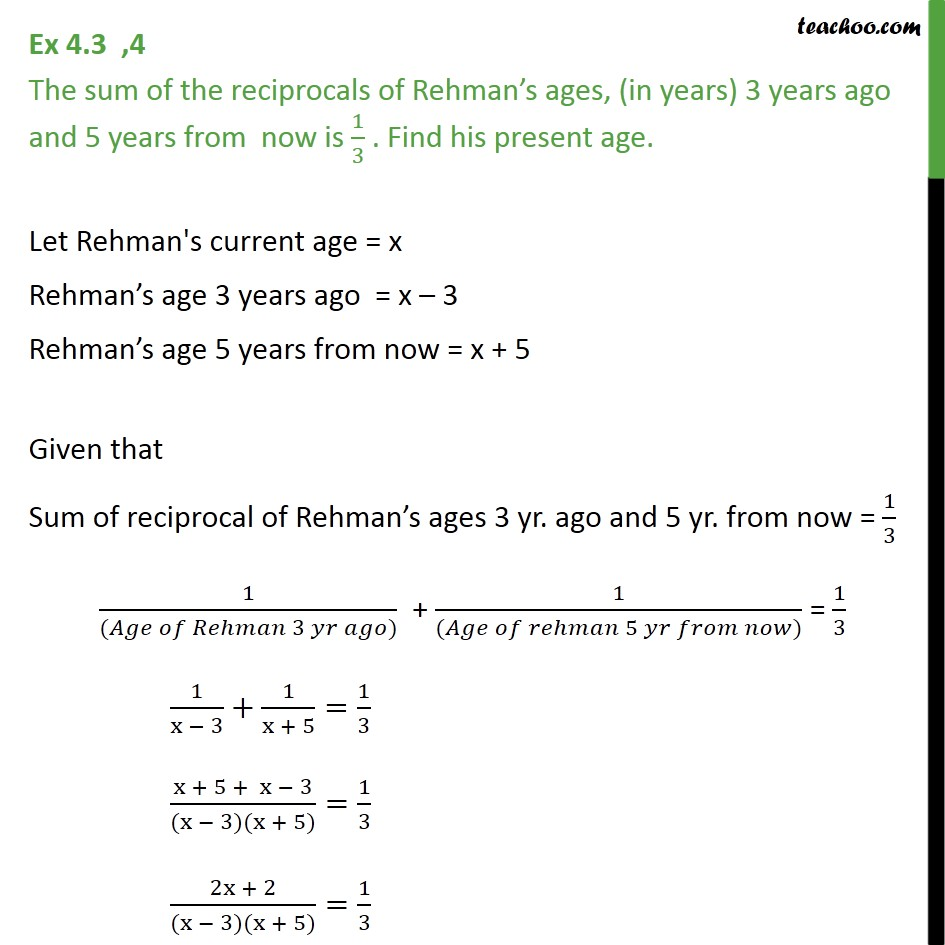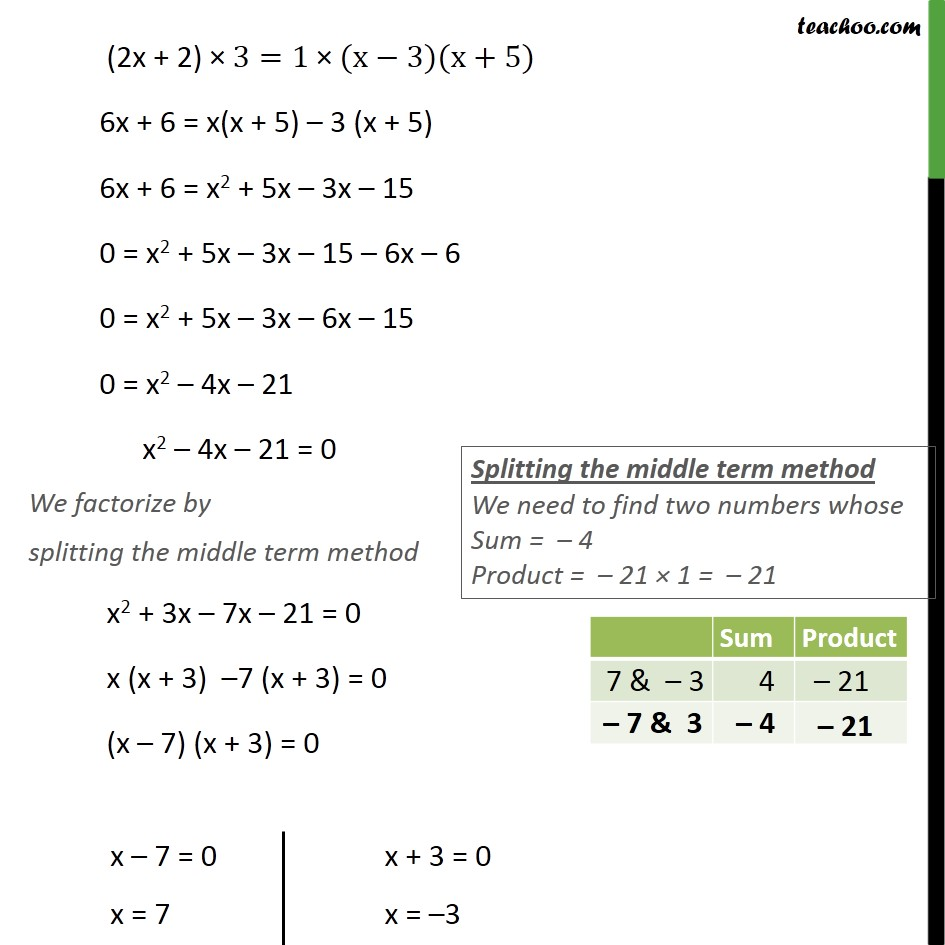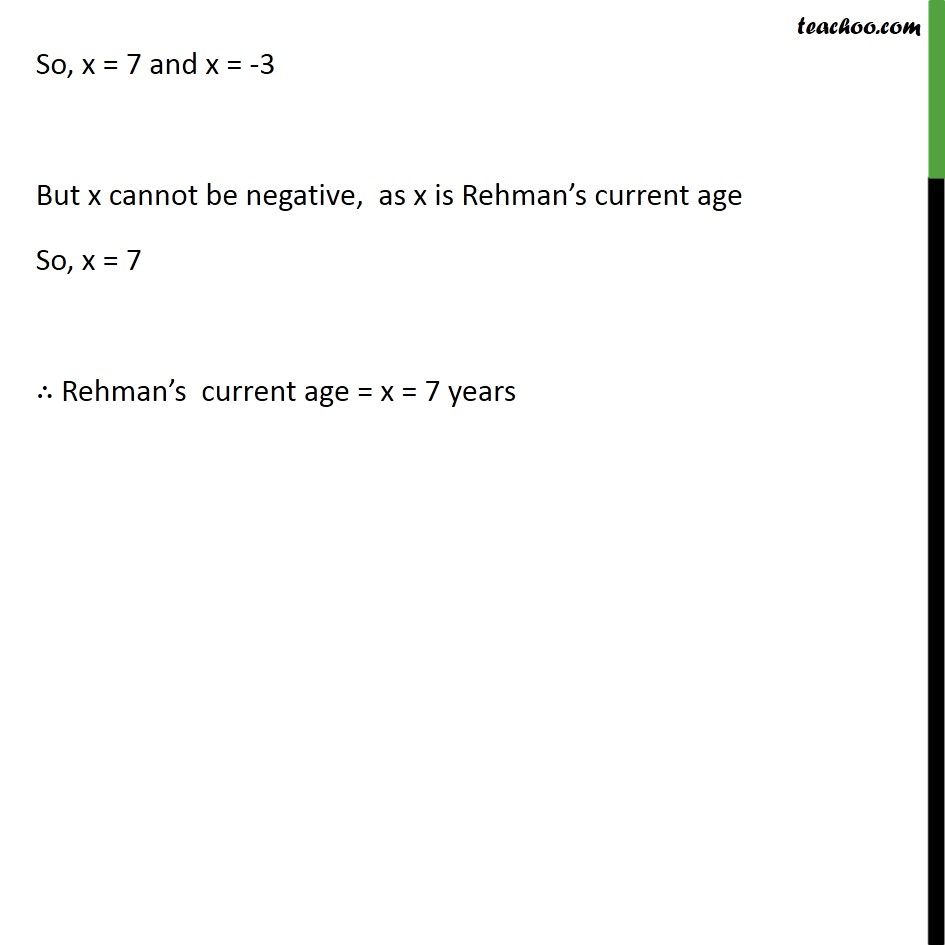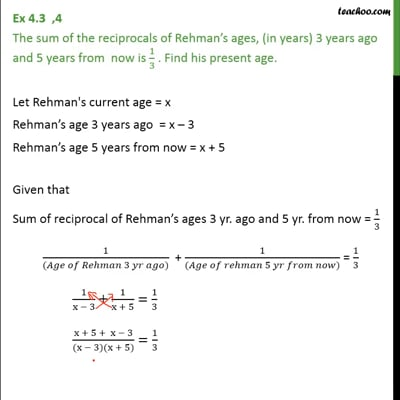Ex 4.3

Chapter 4 Class 10 Quadratic Equations
Serial order wiseThis video is only available for Teachoo black users

Solve all your doubts with Teachoo Black (new monthly pack available now!)

### Transcript

Ex 4.3 ,4 The sum of the reciprocals of Rehman s ages, (in years) 3 years ago and 5 years from now is 1/3 . Find his present age. Let Rehman's current age = x Rehman s age 3 years ago = x 3 Rehman s age 5 years from now = x + 5 Given that Sum of reciprocal of Rehman s ages 3 yr. ago and 5 yr. from now = 1/3 1/(( 3 )) + 1/(( 5 )) = 1/3 1/(x 3)+1/(x + 5)=1/3 (x + 5 + x 3)/((x 3)(x + 5))=1/3 (2x + 2)/((x 3)(x + 5))=1/3 (2x + 2) " " 3=1" " (x 3)(x+5) 6x + 6 = x(x + 5) 3 (x + 5) 6x + 6 = x2 + 5x 3x 15 0 = x2 + 5x 3x 15 6x 6 0 = x2 + 5x 3x 6x 15 0 = x2 4x 21 x2 4x 21 = 0 We factorize by splitting the middle term method x2 + 3x 7x 21 = 0 x (x + 3) 7 (x + 3) = 0 (x 7) (x + 3) = 0 So, x = 7 and x = -3 But x cannot be negative, as x is Rehman s current age So, x = 7 Rehman s current age = x = 7 years